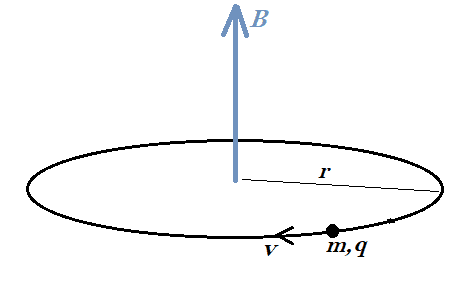• I
hagopbul
TL;DR Summary
read a paper related to electromagnetic force and it mentioned a force i didnt heard before
Hello All:

read a paper related to electromagnetic force and its applications in acceleration of charges particles , some thing came up in it , they drive a force applied on the particle called Faraday force = [1/2B]* [dB/dt]*m*v

B magnetic field , m mass of the particle , v the velocity of the particle

i couldnt find any reference to this force , and didnt help notice the momentum of the particle in the equation

Best
HagopSuppose there is a uniform B field in a region of space and there is a particle of mass ##m## and charge ##q## that circles the magnetic field lines with speed ##v##. The figure is drawn for positive ##q##. For nonrelativistic speeds of the particle, the radius of the orbit is determined to be $$r = \frac{mv}{qB}.$$ There is a flux of magnetic field through the circular path equal to $$\Phi = B \pi r^2.$$ If ##B## starts changing at a rate ##\frac{d B}{dt}##, then there will be an induced emf in the path of the particle equal to $$\varepsilon =\frac{d \Phi}{dt} = \frac{dB}{dt} \pi r^2.$$ The emf is due to an induced electric field ##E## and the direction of ##E## at the location of ##q## will be in the direction of ##\vec v## if ##B## is increasing in strength. The relation between ##\varepsilon## and ##E## is $$\varepsilon = E \cdot 2 \pi r.$$ Thus, there will be an accelerating electric force on the charge given by ##F = qE##.
•